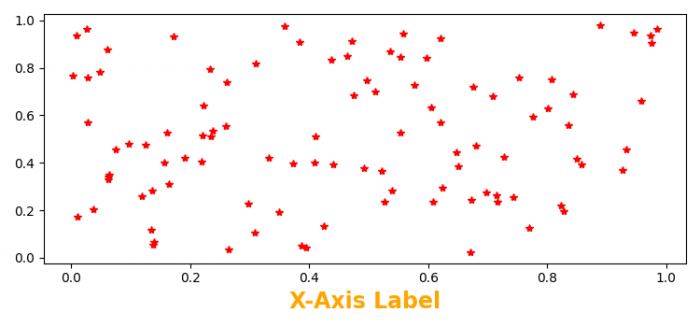# How to customize the X-axis in Matplotlib?

To customize the X-axis label, we can take the following steps −

• Set the figure size and adjust the padding between and around the subplots.
• Initialize a variable, N, to get the number of sample data.
• Create x and y data points using numpy
• Plot x and y data points using plot() method.
• Customize the X-axis labels with fontweight, color, fontsize, and alignment.
• To display the figure, use show() method.

## Example

import numpy as np
import matplotlib.pyplot as plt

plt.rcParams["figure.figsize"] = [7.50, 3.50]
plt.rcParams["figure.autolayout"] = True

N = 100
x = np.random.rand(N)
y = np.random.rand(N)

plt.plot(x, y, 'r*')

plt.xlabel('X-axis Label', fontweight='bold', color='orange',
fontsize='17', horizontalalignment='center')

plt.show()

## Output